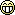# GuideFun with Formations

Hey guys,
There doesn't seem to be a lot of info on formations so I'll post what I came up with today.

I run with 4 bots and here are two macro's i use to align them slightly spread out either EAST/WEST or North/South

2ME N/S
Rich (BB code):
``````/bct toon1 //moveto loc \${Math.Calc[\${Me.Y}-0]} \${Math.Calc[\${Me.X}+10]}
/bct toon2 //moveto loc \${Math.Calc[\${Me.Y}-0]} \${Math.Calc[\${Me.X}+5]}
/bct toon3 //moveto loc \${Math.Calc[\${Me.Y}-0]} \${Math.Calc[\${Me.X}-5]}
/bct toon4 //moveto loc \${Math.Calc[\${Me.Y}-0]} \${Math.Calc[\${Me.X}-10]}``````

2ME E/W
Rich (BB code):
``````/bct toon1 //moveto loc \${Math.Calc[\${Me.Y}+10]} \${Math.Calc[\${Me.X}+0]}
/bct toon2 //moveto loc \${Math.Calc[\${Me.Y}+5]} \${Math.Calc[\${Me.X}+0]}
/bct toon3 //moveto loc \${Math.Calc[\${Me.Y}-5]} \${Math.Calc[\${Me.X}-0]}
/bct toon4 //moveto loc \${Math.Calc[\${Me.Y}-10]} \${Math.Calc[\${Me.X}-0]}``````

Note: I left the -0's there so you could see the syntax.

I hope you guys come up with some cool formations and post them hereAuthor
rogue601
First release
Last update
Rating
0 ratings